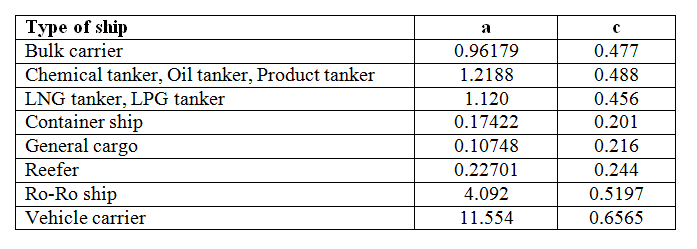# 7.2 IMO data

The IMO data are presented as regression of data for installed engine power for a large number of ships to get specific functions with dwt as the parameter. This can be expressed as CO2 emissions per dwt-tonne and nautical mile as:

3.114 * (0.190 * SPME + 0.210 * SPAE)/(dwt * vref)

Where the factor 3.114 comes from the mass CO2 per mass of fuel used, the factors 0.190 and 0.210 comes from the specific fuel consumption (in kg/kWh) assumed for main engines and auxiliary engines, respectively. PME and PAE are the used power for the main and auxiliary engines. For the main engines this is calculated as 75% of the installed power (MCR). PAE is calculated as 2.5% of the main engine MCR+ 250 kW if MCR > 10 MW, or as 5% of main engine MCR if MCR < 10 MW. vref is the ships’ speed at full load (at dwt) and an engine power of 75% of MCR. vref is based on each ship´s design speed. As we since 2008 have seen a development towards slower speed (slow steam and super slow steam), the model is likely to exaggerate fuel consumption where the ship sail at lower speed than the design speed. NTM currently investigates how this correlation can match reality better.

The equation gives the value of emitted CO2 per dwt-tonne and nautical mile. To convert from nautical mile to km the value should be divided by 1.852.

The regression of the data for a number of ship types to the following type of function:

a * dwt-c

The values of the parameters a and c for the different ship types considered are given in table below (where a is recalculated to kg CO2/dwt -km).Function parameter a and c for different ship types (MEPC 62/24/Add1, MEPC 59/2/23)

The values in above table are expected to be updated in the future. The values for tanker will be given corrections to reflect different densities of the load. The values for Ro-Ro and Vehicle carriers have not been adopted by IMO but are from a working paper. The fleet of Ro-Ro ships show a large spread in data reflecting a relatively large difference in fuel consumption between different Ro-Ro ships as related to transport work. Values for Ro-Ro ships should therefore be used with care. Data for passenger ships are missing.

The function above can be used to calculate the CO2 emissions from the ship per km (ED) as

ED(kg CO2/km) = a * dwt1-c, where ED is a function of dwt and ship type and applies for a fully loaded ship.

The ED can be expressed as mass of fuel rather than CO2 by dividing by 3.114. To get ED in heat content, multiply the latter by 0.041 GJ/kg fuel. Thus

ED(GJ/km) = 0.041/3.114 * a * dwt1-c.